# cs224n自然语言处理公开课笔记（三）

## Lecture 8 - Language Model and RNN

Posted by Yunlongs on October 1, 2019

# Lecture 8 - Language Model and RNN

## 1. Language Model

### 1.1 引言

Language Model 是用来计算一系列单词在一句子中同时出现的概率，通常只依赖于前n个单词：$P\left(w_{1}, \ldots, w_{m}\right)=\prod_{i=1}^{i=m} P\left(w_{i} | w_{1}, \ldots, w_{i-1}\right) \approx \prod_{i=1}^{i=m} P\left(w_{i} | w_{i-n}, \ldots, w_{i-1}\right)$

## 2. Recurrent Neural Networks (RNN)

• Tx: 代表输入序列的长度
• Ty: 代表输出序列的长度
• $x^{\lt t \gt}$： 在第t时间步时的输入
• $\hat y^{\lt t \gt}$： 在第t时间步时的输出

• $W_{ax}$： 管理着从输出$x^{\lt t \gt}$到隐藏层之间的参数
• $W_{aa}$： 管理着两个隐藏层激活值之间的参数
• $W_{ya}$： 管理着每一个隐藏层激活值$a^{\lt t \gt}$到输出层$\hat y^{\lt t \gt}$之间的参数

$a^{\lt 0 \gt}$一般为零向量，全局共享参数意味着每一层的循环神经网络都只有且只需要更新三个参数。

### 2.1 RNN的前向传播

$a^{\lt t \gt}=g_1\left(W_{a a} \cdot a^{\lt t-1 \gt}+W_{a x} \cdot x^{\lt t \gt}+b_{a}\right) \tag {1}$

$\hat{y}^{\lt t \gt}=g_2\left(W_{y a} \cdot a^{\lt t \gt}+b_{y}\right) \tag {2}$ 此处的$g_1$一般取tanh或relu，$g_2$一般取softmax或sigmoid。

Loss Function：（假设是二分类，若是多分类使用softmax损失）

$L^{\lt t \gt}\left(\hat{y}^{\lt t \gt}, y^{\lt t \gt}\right)=-y^{\lt t \gt} \log \hat{y}^{\lt t \gt}-\left(1-y^{\lt t \gt}\right) \log \left(1-\hat{y}^{\lt t \gt}\right) \tag {3}$

cost Funtion： $J(\hat{y}, y)=\sum_{t=1}^{T_{y}} L^{\lt t \gt}\left(\hat{y}^{\lt t \gt}, y^{\lt t \gt}\right) \tag {4}$

### 2.2 RNN的反向传播

$L^{\lt t \gt}\left(\hat{y}^{\lt t \gt}, y^{\lt t \gt}\right)=-y^{\lt t \gt} \log \hat{y}^{\lt t \gt}-\left(1-y^{\lt t \gt}\right) \log \left(1-\hat{y}^{\lt t \gt}\right) \tag {2}$ $\hat{y}^{\lt t \gt}=g_2\left(W_{y a} \cdot a^{\lt t \gt}+b_{y}\right) \tag {3}$ $a^{\lt t \gt}=g_1\left(W_{a a} \cdot a^{\lt t-1 \gt}+W_{a x} \cdot x^{\lt t \gt}+b_{a}\right) \tag {4}$

$\frac {\partial J}{\partial W_{aa}} = \sum_{t=1}^{T_y} \frac {\partial J}{\partial \hat y^{\lt t \gt}} \frac {\partial \hat y^{\lt t \gt}}{\partial a^{\lt t \gt}} \frac{\partial a^{\lt t \gt}}{\partial W_{aa}} = \sum_{t=1}^{T_y} \frac {\partial J}{\partial a^{\lt t \gt}} \frac{\partial a^{\lt t \gt}}{\partial W_{aa}}$

$\frac{\partial J}{\partial a^{\lt t-1 \gt}} = \frac{\partial J}{\partial a^{\lt t \gt}} \frac{\partial a^{\lt t \gt}}{\partial a^{\lt t-1 \gt}}$

$\frac{\partial a^{\lt t \gt}}{\partial a^{\lt t-1 \gt}}=W_{a a}^{T} \cdot\left(1-\tanh \left(W_{ax} x^{\lt t \gt}+W_{aa} a^{\lt t-1 \gt}+b_a\right)^{2}\right)$

$\frac{\partial a^{\lt t \gt}}{\partial W_{aa}}=\left(1-\tanh \left(W_{a x} x^{\lt t \gt}+W_{aa} a^{\lt t-1 \gt}+b\right)^{2}\right) a^{\lt t-1 \gt T}$

2. 对梯度进行L2范数的计算$|g|_ 2=\sqrt{\sum_{i} g_{i}^{2}}$
4. 如果$|g|_ {2}$更大 ，缩放因子Scale $=g r a d_{-} c l i p /|g|$
6. 最后将梯度乘上缩放因子scale 来得到需要的梯度来更新参数

### 2.4 循环神经网络的类型

• 一对一，当去掉$a^{\lt 0 \gt}$时它就是一种标准类型的神经网络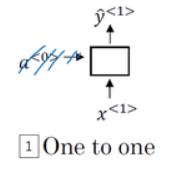• 一对多，比如音乐生成或者序列生成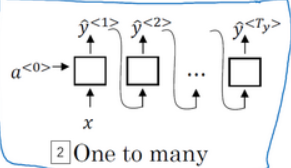• 多对一，如是情感分类的例子，首先读取输入，一个电影评论的文本，然后判断他们是否喜欢电影还是不喜欢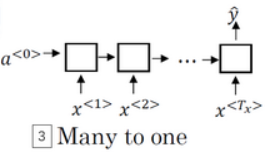• 多对多 ，如命名实体识别，$T_{x}=T_{y}$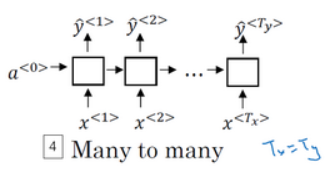• 多对多，如机器翻译，$T_{x} \neq T_{y}$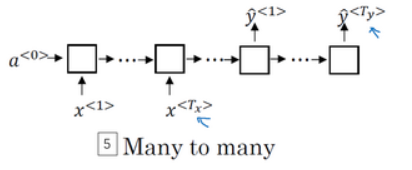## 3. 双向循环神经网络（Bidirectional RNN）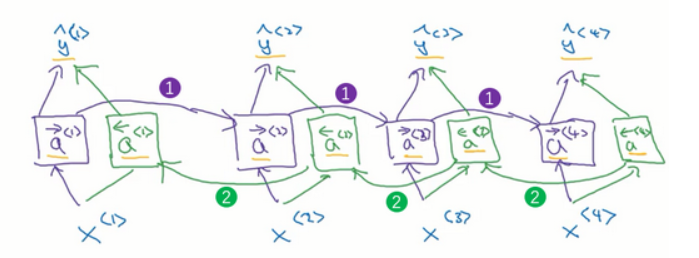$\hat{y}=g\left(W_{g}\left[a^{\rightarrow\lt t \gt}, a^{\leftarrow\lt t \gt}\right]+b_{y}\right)$ BRNN的前向传播过程即需要从左向右计算一次激活值（1），也需要从右向左计算一次激活值（2），只有等每个单元的右向激活值和左向激活值都计算完毕后，才可以进行预测计算$\hat y{\lt t \gt}$。 而图中的每个激活单元可以选用GRU单元也可以选用LSTM单元。

### 4. 深层循环神经网络（Deep RNNs）